Question

# I shoot a basketball that has a mass of 0.5 kg, off a table using the...

I shoot a basketball that has a mass of 0.5 kg, off a table using the spring. The spring constant is 10,000 N/m and the spring is compressed a distance 5 cm before the ball is released.

a) How fast is the ball moving after it leaves the spring?

b) How fast is the ball moving before it hits the ground, which is 1 m below the top of the table?

c)The ball bounces and looses 30% of it’s total energy. What is the max height of the ball after the bounce?

thanks. let me know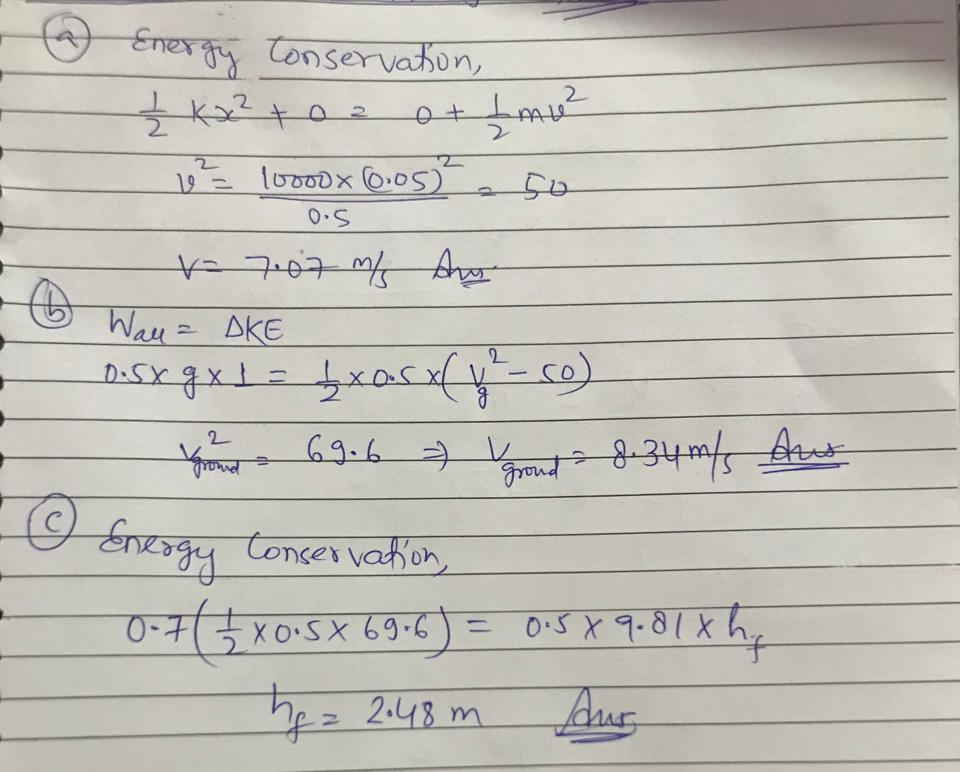#### Earn Coins

Coins can be redeemed for fabulous gifts.

Similar Homework Help Questions
• ### Golf ball and Basketball Collision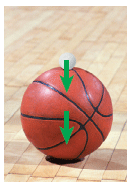Here is a popular lecture demonstration that you can perform at home. Place a golf ball on top of a basketball, and drop the pair from rest so they fall to theground. (For reasons that should become clear once you solve this problem, do not attempt to do this experiment inside, but outdoors instead!) With a littlepractice, you can achieve the situation pictured here: The golf ball stays on top of the basketball until the basketball hits the floor. The...

• ### Problem 5. (20 points) A block of mass 2 kg on top of a table is...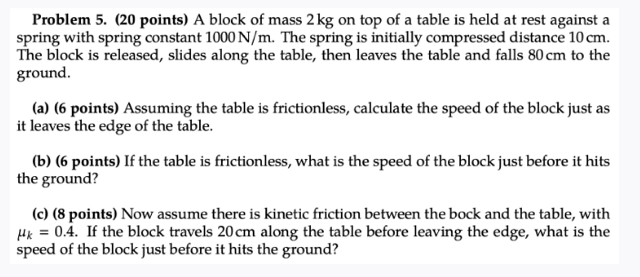Problem 5. (20 points) A block of mass 2 kg on top of a table is held at rest against a spring with spring constant 1000 N/m. The spring is initially compressed distance 10 cm. The block is released, slides along the table, then leaves the table and falls 80 cm to the ground. (a) (6 points) Assuming the table is frictionless, calculate the speed of the block just as it leaves the edge of the table. (b) (6 points)...

• ### Question # 13,15 and 16 s 12) A man drops a 10 kg object from a...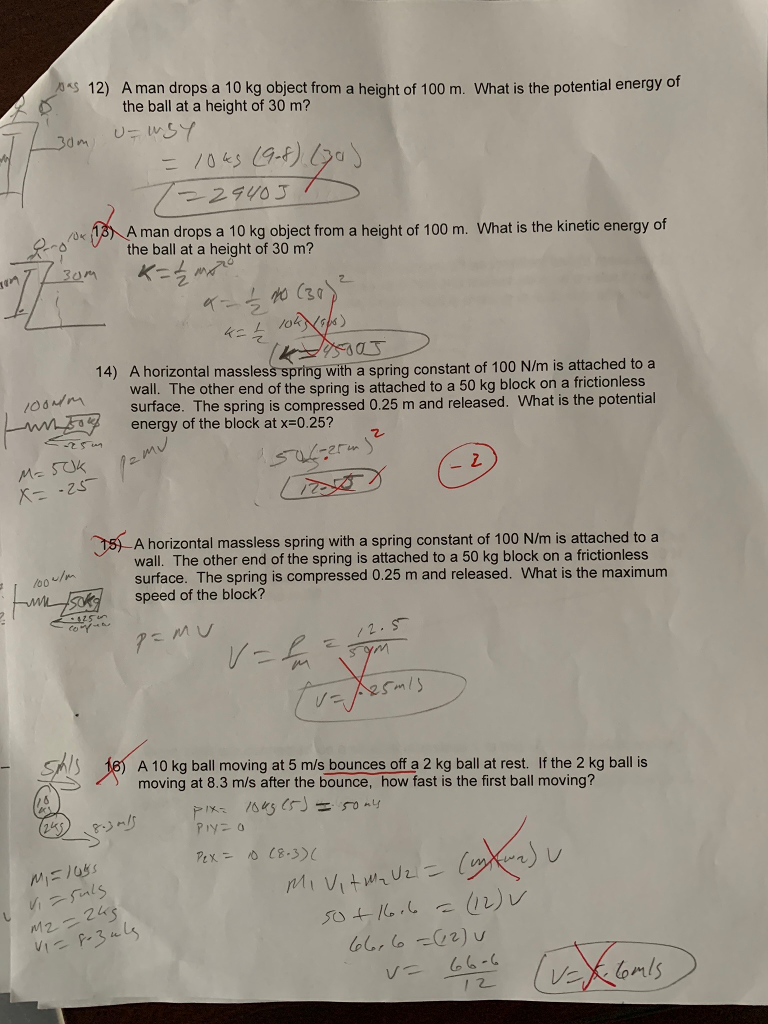Question # 13,15 and 16 s 12) A man drops a 10 kg object from a height of 100 m. What is the potential energy of Dthe ball at a height of 30 m? A man drops a 10 kg object from a height of 100 m. What is the kinetic energy of the ball at a height of 30 m? 2. 2 て 叮 A horizontal massless spring with a spring constant of 100 N/m is attached to a...

• ### Part 1 A ball is tossed so that it bounces off the ground, rises to a...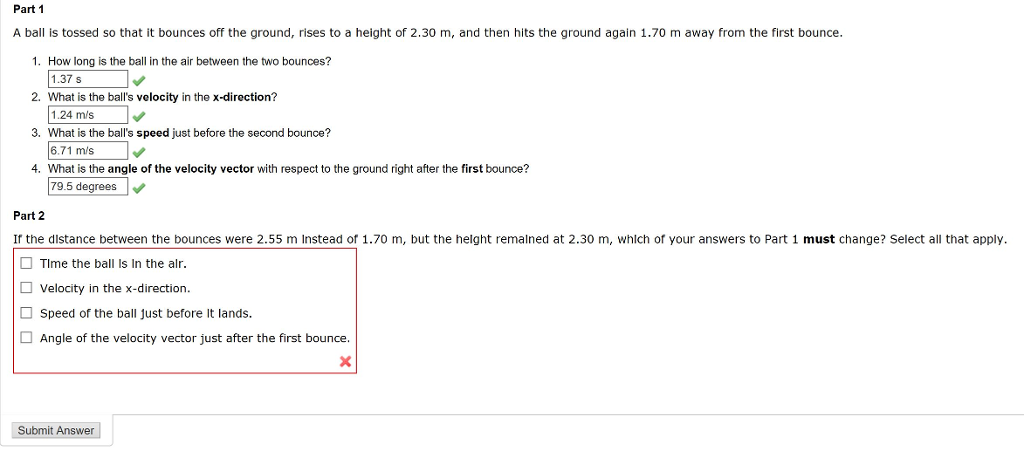Part 1 A ball is tossed so that it bounces off the ground, rises to a height of 2.30 m, and then hits the ground again 1.70 m away from the first bounce. 1. How long is the ball in the air between the two bounces? 1.375 2. What is the ball's velocity in the x-direction? 3. What is the ball's speed just before the second bounce? 4. What is the angle of the velocity vector with respect to the...

• ### Ball A (m 0.5 kg, I 0.7 MR2) is held at rest against a spring, compressed by a distance x-40 cm. The ball is released...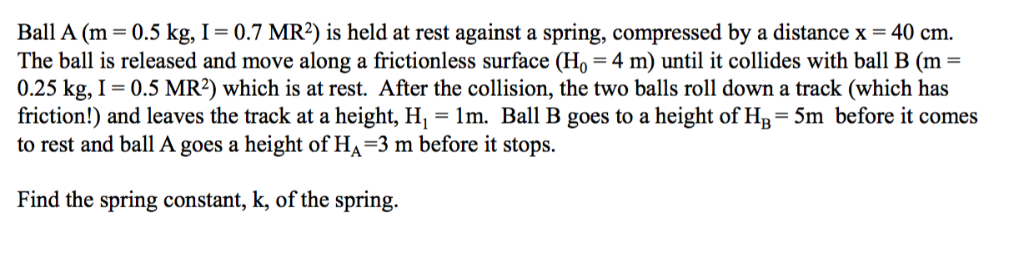Ball A (m 0.5 kg, I 0.7 MR2) is held at rest against a spring, compressed by a distance x-40 cm. The ball is released and move along a frictionless surface (Ho 4 m) until it collides with ball B (m- 0.25 kg, I- 0.5 MR2) which is at rest. After the collision, the two balls roll down a track (which has friction!) and leaves the track at a height, H,- 1m. Ball B goes to a height of HB-5m...

• ### Springing Off the Table Due this Friday, Nov 13 at 11:59 pm (EST) A 0.272-kg box...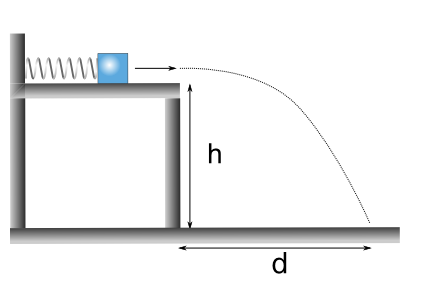Springing Off the Table Due this Friday, Nov 13 at 11:59 pm (EST) A 0.272-kg box is placed in contact with a spring of stiffness 4.352×102 N/m. The spring is compressed 1.08×10-1 m from its unstrained length. The spring is then released, the block slides across a frictionless tabletop, and it flies through the air. The tabletop is a height of 1.060 m above the floor. [mass_spring_table] (the drawing is not to scale) What is the potential energy stored in...

• ### A 1 kg ball bounces off the ground as shown: 45° The ball's kinetic energy immediately...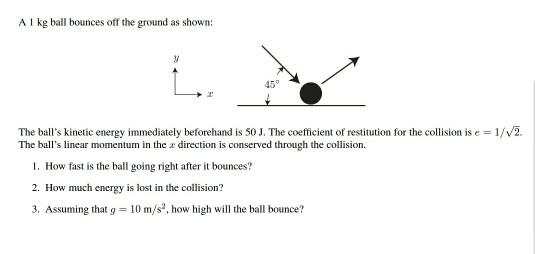A 1 kg ball bounces off the ground as shown: 45° The ball's kinetic energy immediately beforehand is 50 J. The coefficient of restitution for the collision is e = 1/V2. The ball's linear momentum in the r direction is conserved through the collision 1. How fast is the ball going right after it bounces? 2. How much energy is lost in the collision? 3, Assuming that g = 10 m/s2, how high will the ball bounce?

• ### 1. A ping pong ball (ball #1, mass of 2g) is held just above a tennis...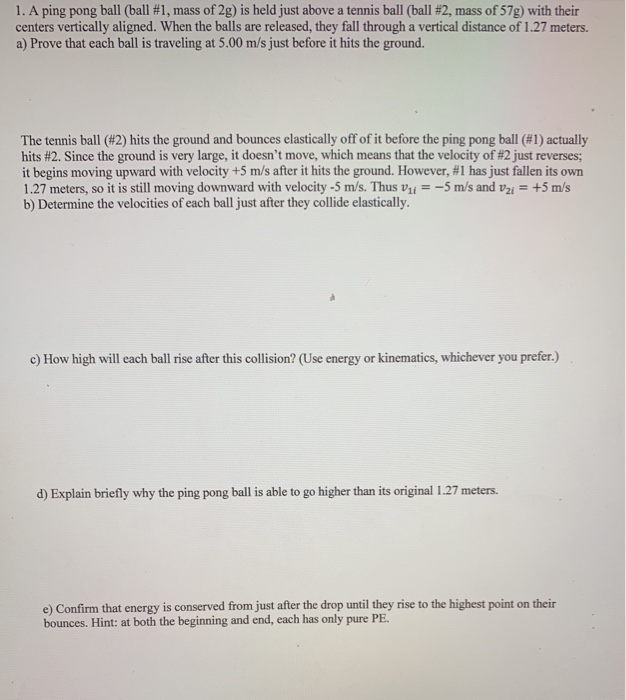1. A ping pong ball (ball #1, mass of 2g) is held just above a tennis ball (ball #2, mass of 57g) with their centers vertically aligned. When the balls are released, they fall through a vertical distance of 1.27 meters. a) Prove that each ball is traveling at 5.00 m/s just before it hits the ground. The tennis ball (#2) hits the ground and bounces elastically off of it before the ping pong ball (#1) actually hits #2. Since...

• ### A tennis ball of mass 0.063kg is held just above a basketball of mass 0.530kg. With...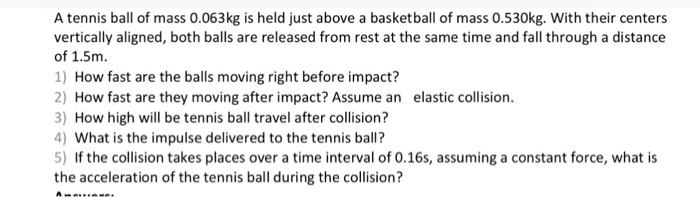A tennis ball of mass 0.063kg is held just above a basketball of mass 0.530kg. With their centers vertically aligned, both balls are released from rest at the same time and fall through a distance of 1.5m. 1) How fast are the balls moving right before impact? 2) How fast are they moving after impact? Assume an elastic collision 3) How high will be tennis ball travel after collision? ) What is the impulse delivered to the tennis ball? 5)...

• ### A student pushes a baseball of m = 0.11 kg down onto the top of a vertical spring that has its lower end fixed table

A student pushes a baseball of m = 0.11 kg down onto the top of a vertical spring that has its lower end fixed table, compressing the spring a distance of d = 0.12 meters. The spring constant of the spring is k = 580 N/m. Let the gravity potential energy be zero at the position of the baseball in the compressed spring. Part (a) The ball is then released. What is its speed, v, in meters per second, just after...